# 欢迎关注WX公众号：【程序员管小亮】

## 一、你好世界Python是一种现代的，易于学习的面向对象的编程语言。 它具有一组强大的内置数据类型和易于使用的控件结构。 由于是解释型语言，因此通过简单地查看和描述交互式会话，更容易进行检查。所以好多人会和你说推荐你使用 anaconda 的，比如：深度学习入门笔记（五）：神经网络的编程基础

jupyter notebook>>> 是提示输入语句，然后计算你提供的Python语句。 例如：

>>> print("Hello，World")
Hello，World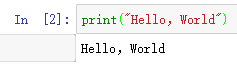>>> print("\n".join("Hello World"))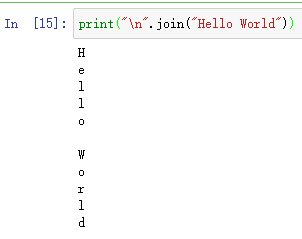## 二、数据入门Python有：

• 两个主要的内置数字类，分别是 int （整型数据类型）和 float（浮点数据类型）。
• 标准的算术运算，+-*/，和 **（取幂），可以用括号强制操作的顺序来规避正常的操作符优先级。
• 其他很有用的操作是 余数（模组）操作符%、和 整数除法//。注意，当两个整数相除，结果是一个浮点数。整数除法运算符通过截断所有小数部分来返回商的整数部分。
• 布尔数据类型，作为Python bool类的实现，在表示真值时非常有用。

>>> True
True

>>> False
False

>>> False or True
True

>>> not (False or True)
False

>>> True and True
True>>> theSum = 0
>>> theSum
0

>>> theSum = theSum + 1
>>> theSum
1

>>> theSum = True
>>> theSum
True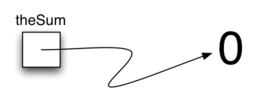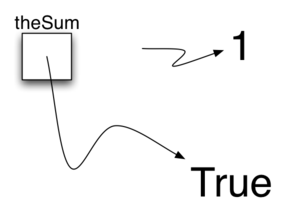• 列表、字符串和元组是有序集合。
• 集合和字典是无序的集合。

#### 2.1、列表

>>> [1,3,True,6.5]
[1, 3, True, 6.5]

>>> myList = [1,3,True,6.5]
>>> myList
[1, 3, True, 6.5]>>> myList =  * 6
>>> myList
[0, 0, 0, 0, 0, 0]


>>> myList = [1,2,3,4]
>>> A = [myList]*3
>>> print(A)
[[1, 2, 3, 4], [1, 2, 3, 4], [1, 2, 3, 4]]

>>> myList=45
>>> print(A)
[[1, 2, 45, 4], [1, 2, 45, 4], [1, 2, 45, 4]]>>> myList = [1024, 3, True, 6.5]
>>> myList.append(False)
>>> print(myList)
[1024, 3, True, 6.5, False]

>>> myList.insert(2,4.5)
>>> print(myList)
[1024, 3, 4.5, True, 6.5, False]

>>> print(myList.pop())
False

>>> print(myList)
[1024, 3, 4.5, True, 6.5]

>>> print(myList.pop(1))
3

>>> print(myList)
[1024, 4.5, True, 6.5]

>>> myList.pop(2)
>>> print(myList)
[1024, 4.5, 6.5]


>>> myList.sort()
>>> print(myList)
[4.5, 6.5, 1024]

>>> myList.reverse()
>>> print(myList)
[1024, 6.5, 4.5]

>>> print(myList.count(6.5))
1
>>> print(myList.index(4.5))
2

>>> myList.remove(6.5)
>>> print(myList)
[1024, 4.5]

>>> del myList
>>> print(myList)
[4.5]


>>> (54).__add__(21)
75
>>> 54+21
75


range函数

>>> range(10)
range(0, 10)
>>> list(range(10))
[0, 1, 2, 3, 4, 5, 6, 7, 8, 9]

>>> range(5,10)
range(5, 10)
>>> list(range(5,10))
[5, 6, 7, 8, 9]

>>> list(range(5,10,2))
[5, 7, 9]

>>> list(range(10,1,-1))
[10, 9, 8, 7, 6, 5, 4, 3, 2]


range 对象代表一个整数序列。默认情况下，它将从0开始。常用的参数有三个，在特定的点开始和结束，甚至可以跳过某项。

• 在第一个例子中，（10），序列从0开始，递增到10但不包括10。
• 在第二个例子中，范围（5,10）从5开始，递增到10但不包括10。
• 在第三个例子中，范围（5,10,2）类似的执行，但是跳过2（同样，10不包括在内）。

#### 2.2、字符串

>>> "David"
'David'
>>> myName = "David"
>>> myName
'i'
>>> myName*2
'DavidDavid'
>>> len(myName)
5>>> myName
'David'
>>> myName.upper()
'DAVID'
>>> myName.center(10)
'  David   '
>>> myName.find('v')
2

>>> myName.split('v')
['Da', 'id']
>>> myName.split()
['David']


>>> myList
[1, 3, True, 6.5]
>>> myList=2**10
>>> myList
[1024, 3, True, 6.5]

>>> myName
'David'
>>> myName='X'
---------------------------------------------------------------------------
TypeError                                 Traceback (most recent call last)
<ipython-input-7-c44c71c6d2f3> in <module>
1 myName = "David"
----> 2 myName='X'

TypeError: 'str' object does not support item assignment


#### 2.3、元组

>>> myTuple = (2,True,4.96)
>>> myTuple
(2, True, 4.96)
>>> len(myTuple)
3
>>> myTuple
2
>>> myTuple * 3
(2, True, 4.96, 2, True, 4.96, 2, True, 4.96)
>>> myTuple[0:2]
(2, True)


>>> myTuple = 1
---------------------------------------------------------------------------
TypeError                                 Traceback (most recent call last)
<ipython-input-6-a2c5dba3de3b> in <module>
1 myTuple = (2,True,4.96)
----> 2 myTuple = 1

TypeError: 'tuple' object does not support item assignment


#### 2.4、集合

set 是一个无序的， 为空或是更多不可变的Python数据对象集合。集合中的值不允许重复，写在大括号中。空集合由 set() 表示。如下所示。

>>> {3,6,"cat",4.5,False}
{False, 4.5, 3, 6, 'cat'}
>>> mySet = {3,6,"cat",4.5,False}
>>> mySet
{False, 4.5, 3, 6, 'cat'}
>>>>>> mySet
{False, 4.5, 3, 6, 'cat'}
>>> len(mySet)
5
>>> False in mySet
True
>>> "dog" in mySet
False>>> mySet
{False, 4.5, 3, 6, 'cat'}
>>> yourSet = {99,3,100}
>>> mySet.union(yourSet)
{False, 4.5, 3, 100, 6, 'cat', 99}
>>> mySet | yourSet
{False, 4.5, 3, 100, 6, 'cat', 99}
>>> mySet.intersection(yourSet)
{3}
>>> mySet & yourSet
{3}
>>> mySet.difference(yourSet)
{False, 4.5, 6, 'cat'}
>>> mySet - yourSet
{False, 4.5, 6, 'cat'}
>>> {3,100}.issubset(yourSet)
True
>>> {3,100}<=yourSet
True

>>> mySet.add("house")
>>> mySet
{False, 4.5, 3, 6, 'house', 'cat'}
>>> mySet.remove(4.5)
>>> mySet
{False, 3, 6, 'house', 'cat'}
>>> mySet.pop()
False
>>> mySet
{3, 6, 'house', 'cat'}
>>> mySet.clear()
>>> mySet
set()


#### 2.5、字典

>>> capitals = {'Iowa':'DesMoines','Wisconsin':'Madison'}
>>> capitals


>>> capitals = {'Iowa':'DesMoines','Wisconsin':'Madison'}
>>> print(capitals['Iowa'])
DesMoines
>>> capitals['Utah']='SaltLakeCity'
>>> print(capitals)
{'Iowa': 'DesMoines', 'Wisconsin': 'Madison', 'Utah': 'SaltLakeCity'}
>>> capitals['California']='Sacramento'
>>> print(capitals)
{'Iowa': 'DesMoines', 'Wisconsin': 'Madison', 'Utah': 'SaltLakeCity', 'California': 'Sacramento'}
>>> print(len(capitals))
4

>>> for k in capitals:
print(capitals[k]," is the capital of ", k)
DesMoines  is the capital of  Iowa
Madison  is the capital of  Wisconsin
SaltLakeCity  is the capital of  Utah
Sacramento  is the capital of  California>>> phoneext={'david':1410,'brad':1137}
>>> phoneext
>>> phoneext.keys()
>>> list(phoneext.keys())
>>> phoneext.values()
dict_values([1137, 1410])
>>> list(phoneext.values())
[1137, 1410]
>>> phoneext.items()
>>> list(phoneext.items())


keysvaluesitems 方法都返回包含感兴趣的值的对象。而 get 方法有两种变体，如果字典里没有对应键，get 将返回空。然而，第二个可选参数可以指定一个返回值。>>> phoneext={'david':1410,'brad':1137}
>>> phoneext
>>> phoneext.get("kent")
# 返回空说明没有对应的键
>>> phoneext.get("kent","NO ENTRY")
'NO ENTRY'


## 三、输入和输出>>> aName = input('Please enter your name: ')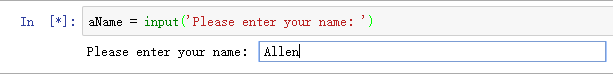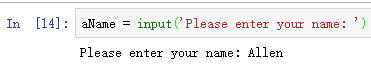>>> aName = input("Please enter your name ")
print("Your name in all capitals is",aName.upper(),
"and has length", len(aName))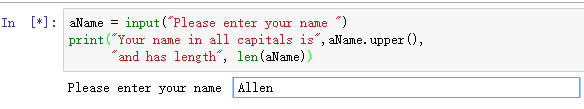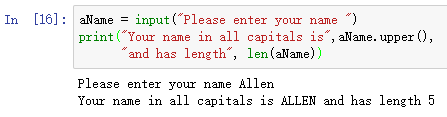>>> sradius = input("Please enter the radius of the circle ")
print(diameter)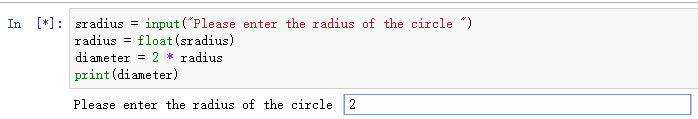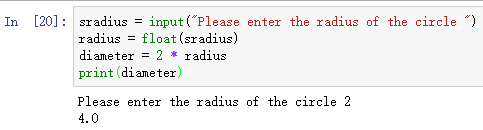>>> print("Hello")
Hello
>>> print("Hello","World")
Hello World
>>> print("Hello","World", sep="***")
Hello***World
>>> print("Hello","World", end="***")
Hello World***


Python提供了一种称为格式化字符串的替代方案。格式化的字符串是一个模板，在这个模板中，结合了保持不变的单词或空格与插入到字符串中的变量的占位符。例如：

>>> print(aName, "is", age, "years old.")


print("%s is %d years old." % (aName, age))• 如果是一个元组，那么这些值就会按照位置的顺序插入；
• 如果集合是一个字典，那么这些值就会按照键插入键所对应的值。在这种情况下，所有格式字符都必须使用(name)修饰符来指定键的名称。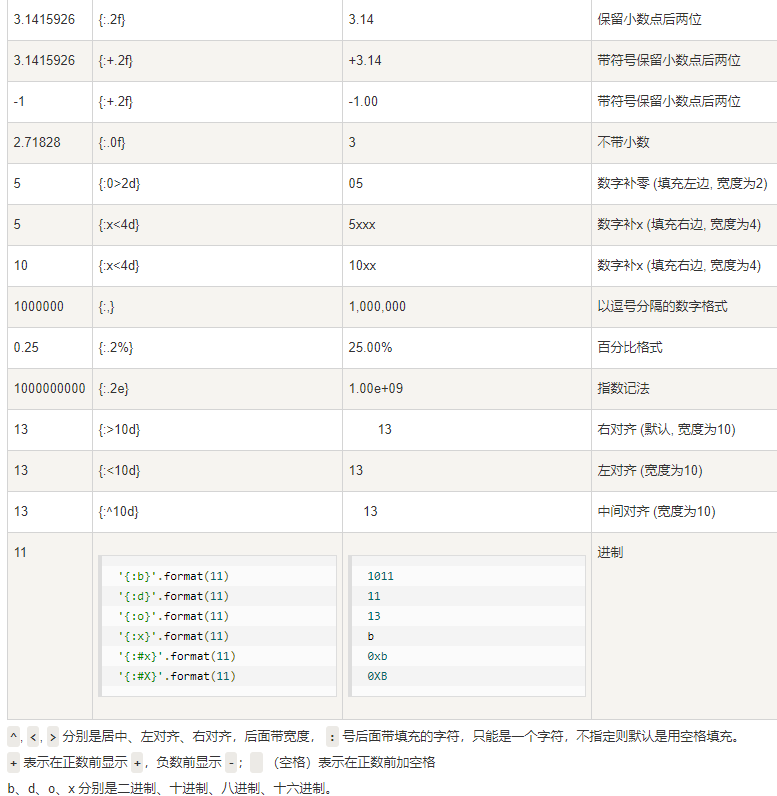>>> price = 24
>>> item = "banana"
>>> print("The %s costs %d cents"%(item,price))
The banana costs 24 cents
>>> print("The %+10s costs %5.2f cents"%(item,price))
The     banana costs 24.00 cents
>>> print("The %+10s costs %10.2f cents"%(item,price))
The     banana costs      24.00 cents

>>> itemdict = {"item":"banana","cost":24}
>>> print("The %(item)s costs %(cost)7.1f cents"%itemdict)
The banana costs    24.0 cents


>>> print("{} {}".format("hello", "world"))
hello world
>>> print("{0} {1}".format("hello", "world"))
hello world
>>> print("{1} {0} {1}".format("hello", "world"))
world hello world


## 四、控制结构

>>> counter = 1
>>> while counter <= 5:
...     print("Hello, world")
...     counter = counter + 1

Hello, world
Hello, world
Hello, world
Hello, world
Hello, world


while 语句是一个非常通用的迭代结构，在许多情况下，复合条件将控制迭代。

while counter <= 10 and not done:
...
...


>>> for item in [1,3,6,2,5]:
...    print(item)
1
3
6
2
5


for 语句的一个常见用途是在一系列值上实现明确的迭代。 如下

>>> for item in range(5):
...    print(item**2)
...
0
1
4
9
16
>>>


>>> wordlist = ['cat','dog','rabbit']
letterlist = [ ]
for aword in wordlist:
for aletter in aword:
letterlist.append(aletter)
print(letterlist)
['c', 'a', 't', 'd', 'o', 'g', 'r', 'a', 'b', 'b', 'i', 't']


if score >= 90:
print('A')
else:
if score >=80:
print('B')
else:
if score >= 70:
print('C')
else:
if score >= 60:
print('D')
else:
print('F')


if score >= 90:
print('A')
elif score >=80:
print('B')
elif score >= 70:
print('C')
elif score >= 60:
print('D')
else:
print('F')


>>> sqlist=[]
>>> for x in range(1,11):
sqlist.append(x*x)
>>> sqlist
[1, 4, 9, 16, 25, 36, 49, 64, 81, 100]


>>> sqlist=[x*x for x in range(1,11)]
>>> sqlist
[1, 4, 9, 16, 25, 36, 49, 64, 81, 100]


>>> sqlist=[x*x for x in range(1,11) if x%2 != 0]
>>> sqlist
[1, 9, 25, 49, 81]


>>>[ch.upper() for ch in 'comprehension' if ch not in 'aeiou']
['C', 'M', 'P', 'R', 'H', 'N', 'S', 'N']


## 五、处理异常

• 第一个类型的错误称为 语法错误，仅仅意味着程序员在语句或表达式的结构中犯了一个错误。

>>> for i in range(10)
File "<ipython-input-8-9bf3d452bb2a>", line 1
for i in range(10)
^
SyntaxError: invalid syntax


• 另一种类型的错误称为 逻辑错误，表示程序执行但给出错误结果的情况。 这可能是由于基础算法中的错误或该算法的转换错误。

>>> anumber = int(input("Please enter an integer "))# 输入 -23
>>> import math
>>> print(math.sqrt(anumber))
---------------------------------------------------------------------------
ValueError                                Traceback (most recent call last)
<ipython-input-11-3c97b1b97120> in <module>
1 import math
----> 2 print(math.sqrt(anumber))

ValueError: math domain error


>>> try:
print(math.sqrt(anumber))
except:
print(math.sqrt(abs(anumber)))

4.79583152331


>>> if anumber < 0:
...    raise RuntimeError("You can't use a negative number")
... else:
...    print(math.sqrt(anumber))
---------------------------------------------------------------------------
RuntimeError                              Traceback (most recent call last)
<ipython-input-18-424977cff197> in <module>
1 if anumber < 0:
----> 2     raise RuntimeError("You can't use a negative number")
3 else:
4     print(math.sqrt(anumber))

RuntimeError: You can't use a negative number


BaseException 所有异常的基类
SystemExit解释器请求退出
KeyboardInterrupt 用户中断执行(通常是输入^C)
Exception常规错误的基类
StopIteration 迭代器没有更多的值
GeneratorExit 生成器(generator)发生异常来通知退出
StandardError 所有的内建标准异常的基类
ArithmeticError 所有数值计算错误的基类
FloatingPointError 浮点计算错误
OverflowError 数值运算超出最大限制
ZeroDivisionError 除(或取模)零 (所有数据类型)
AssertionError 断言语句失败
AttributeError 对象没有这个属性
EOFError 没有内建输入,到达EOF 标记
EnvironmentError 操作系统错误的基类
IOError 输入/输出操作失败
OSError 操作系统错误
WindowsError 系统调用失败
ImportError 导入模块/对象失败
LookupError 无效数据查询的基类
IndexError 序列中没有此索引(index)
KeyError 映射中没有这个键
MemoryError 内存溢出错误(对于Python 解释器不是致命的)
NameError 未声明/初始化对象 (没有属性)
UnboundLocalError 访问未初始化的本地变量
ReferenceError 弱引用(Weak reference)试图访问已经垃圾回收了的对象
RuntimeError 一般的运行时错误
NotImplementedError 尚未实现的方法
SyntaxErrorPython 语法错误
IndentationError 缩进错误
TabError Tab 和空格混用
SystemError 一般的解释器系统错误
TypeError 对类型无效的操作
ValueError 传入无效的参数
UnicodeError Unicode 相关的错误
UnicodeDecodeError Unicode 解码时的错误
UnicodeEncodeError Unicode 编码时错误
UnicodeTranslateError Unicode 转换时错误
Warning 警告的基类
DeprecationWarning 关于被弃用的特征的警告
FutureWarning 关于构造将来语义会有改变的警告
OverflowWarning 旧的关于自动提升为长整型(long)的警告
PendingDeprecationWarning 关于特性将会被废弃的警告
RuntimeWarning 可疑的运行时行为(runtime behavior)的警告
SyntaxWarning 可疑的语法的警告
UserWarning 用户代码生成的警告

## 六、定义函数

>>> def square(n):
...    return n**2
>>> square(3)
9
>>> square(square(3))
81


def squareroot(n):
root = n/2    #initial guess will be 1/2 of n
for k in range(20):
root = (1/2)*(root + (n / root))

return root


>>>squareroot(9)
3.0
>>>squareroot(4563)
67.549981495186216


## 七、面向对象编程：定义类

Python是一种 面向对象 的编程语言，面向对象编程语言中最强大的功能之一是允许程序员（问题解决者）创建新类，以模拟解决问题所需的数据。

#### 7.1、一个分数类

class Fraction:

#the methods go here


class Fraction:

def __init__(self,top,bottom):
self.num = top
self.den = bottom


myfraction = Fraction(3,5)>>> myf = Fraction(3,5)
>>> print(myf)
<__main__.Fraction instance at 0x409b1acc>


fraction 对象 myf 不知道如何响应此打印请求。 print 函数要求对象将自身转换为字符串，以便可以将字符串写入输出。 myf 唯一的选择是显示存储在变量中的实际引用（地址本身），然而很明显这不是我们想要的。

def show(self):
print(self.num,"/",self.den)

>>> myf = Fraction(3,5)
>>> myf.show()
3 / 5
>>> print(myf)
<__main__.Fraction instance at 0x40bce9ac>


def __str__(self):
return str(self.num)+"/"+str(self.den)

>>> myf = Fraction(3,5)
>>> print(myf)
3/5
>>> print("I ate", myf, "of the pizza")
I ate 3/5 of the pizza
>>> myf.__str__()
'3/5'
>>> str(myf)
'3/5'


>>> f1 = Fraction(1,4)
>>> f2 = Fraction(1,2)
>>> f1+f2
---------------------------------------------------------------------------
TypeError                                 Traceback (most recent call last)
<ipython-input-20-0210d543cddb> in <module>
1 f1 = Fraction(1,4)
2 f2 = Fraction(1,2)
----> 3 f1+f2

TypeError: unsupported operand type(s) for +: 'Fraction' and 'Fraction'


f1.__add__(f2)


def __add__(self,otherfraction):

newnum = self.num*otherfraction.den + self.den*otherfraction.num
newden = self.den * otherfraction.den

return Fraction(newnum,newden)

>>> f1=Fraction(1,4)
>>> f2=Fraction(1,2)
>>> f3=f1+f2
>>> print(f3)
6/8


def gcd(m,n):
while m%n != 0:
oldm = m
oldn = n

m = oldn
n = oldm%oldn
return n

print(gcd(20,10))


def __add__(self,otherfraction):
newnum = self.num*otherfraction.den + self.den*otherfraction.num
newden = self.den * otherfraction.den
common = gcd(newnum,newden)
return Fraction(newnum//common,newden//common)

>>> f1=Fraction(1,4)
>>> f2=Fraction(1,2)
>>> f3=f1+f2
>>> print(f3)
3/4Fraction 类中，可以通过再次将两个分数放在通用项中然后比较分子来实现 __eq__ 方法，代码如下：

def __eq__(self, other):
firstnum = self.num * other.den
secondnum = other.num * self.den

return firstnum == secondnum


def gcd(m,n):
while m%n != 0:
oldm = m
oldn = n

m = oldn
n = oldm%oldn
return n

class Fraction:
def __init__(self,top,bottom):
self.num = top
self.den = bottom

def __str__(self):
return str(self.num)+"/"+str(self.den)

def show(self):
print(self.num,"/",self.den)

newnum = self.num*otherfraction.den + \
self.den*otherfraction.num
newden = self.den * otherfraction.den
common = gcd(newnum,newden)
return Fraction(newnum//common,newden//common)

def __eq__(self, other):
firstnum = self.num * other.den
secondnum = other.num * self.den

return firstnum == secondnum

x = Fraction(1,2)
y = Fraction(2,3)
print(x+y)
print(x == y)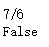#### 7.2、逻辑门和电路• 与门有两条输入线，每条输入线可以是0或1（分别代表False或True）。 如果两个输入行的值都为1，则结果输出为1。但是，如果输入行中的任何一个或两个为0，则结果为0。
• 或门也有两个输入行，如果一个或两个输入行为1，则输出值为1。
• 非门与其他两个门的不同之处在于它们只有一条输入线，输出值与输入值完全相反。 如果输入出现0，则在输出上产生1；类似地，1产生0。class LogicGate:

def __init__(self,n):
self.label = n
self.output = None

def getLabel(self):
return self.label

def getOutput(self):
self.output = self.performGateLogic()
return self.output


class BinaryGate(LogicGate):

def __init__(self,n):
LogicGate.__init__(self,n)

self.pinA = None
self.pinB = None

def getPinA(self):
return int(input("Enter Pin A input for gate "+ self.getLabel()+"-->"))

def getPinB(self):
return int(input("Enter Pin B input for gate "+ self.getLabel()+"-->"))

class UnaryGate(LogicGate):

def __init__(self,n):
LogicGate.__init__(self,n)

self.pin = None

def getPin(self):
return int(input("Enter Pin input for gate "+ self.getLabel()+"-->"))


BinaryGate 类添加的唯一行为是从两个输入行获取值的能力。 由于这些值来自某些外部位置，只需通过输入语句询问用户即可。 除了只有一个输入行之外，UnaryGate 类也会进行相同的实现。

class AndGate(BinaryGate):

def __init__(self,n):
BinaryGate.__init__(self,n)

def performGateLogic(self):

a = self.getPinA()
b = self.getPinB()
if a==1 and b==1:
return 1
else:
return 0


AndGate 唯一需要添加的是执行前面描述的布尔操作的特定行为。 这是可以提供 performGateLogic 方法的地方。 对于 AND 门，此方法首先必须获取两个输入值，然后仅在两个输入值均为1时返回1。完整类如下所示。

>>> g1 = AndGate("G1")
>>> g1.getOutput()
Enter Pin A input for gate G1-->1
Enter Pin B input for gate G1-->0
0


>>> g2 = OrGate("G2")
>>> g2.getOutput()
Enter Pin A input for gate G2-->1
Enter Pin B input for gate G2-->1
1
>>> g2.getOutput()
Enter Pin A input for gate G2-->0
Enter Pin B input for gate G2-->0
0
>>> g3 = NotGate("G3")
>>> g3.getOutput()
Enter Pin input for gate G3-->0
1Connector类的代码如下。

class Connector:

def __init__(self, fgate, tgate):
self.fromgate = fgate
self.togate = tgate

tgate.setNextPin(self)

def getFrom(self):
return self.fromgate

def getTo(self):
return self.togate


def setNextPin(self,source):
if self.pinA == None:
self.pinA = source
else:
if self.pinB == None:
self.pinB = source
else:
raise RuntimeError("Error: NO EMPTY PINS")


def getPinA(self):
if self.pinA == None:
return input("Enter Pin A input for gate " + self.getName()+"-->")
else:
return self.pinA.getFrom().getOutput()


>>> g1 = AndGate("G1")
>>> g2 = AndGate("G2")
>>> g3 = OrGate("G3")
>>> g4 = NotGate("G4")
>>> c1 = Connector(g1,g3)
>>> c2 = Connector(g2,g3)
>>> c3 = Connector(g3,g4)


>>> g4.getOutput()
Pin A input for gate G1-->0
Pin B input for gate G1-->1
Pin A input for gate G2-->1
Pin B input for gate G2-->1
0


class LogicGate:

def __init__(self,n):
self.name = n
self.output = None

def getName(self):
return self.name

def getOutput(self):
self.output = self.performGateLogic()
return self.output

class BinaryGate(LogicGate):

def __init__(self,n):
LogicGate.__init__(self,n)

self.pinA = None
self.pinB = None

def getPinA(self):
if self.pinA == None:
return int(input("Enter Pin A input for gate "+self.getName()+"-->"))
else:
return self.pinA.getFrom().getOutput()

def getPinB(self):
if self.pinB == None:
return int(input("Enter Pin B input for gate "+self.getName()+"-->"))
else:
return self.pinB.getFrom().getOutput()

def setNextPin(self,source):
if self.pinA == None:
self.pinA = source
else:
if self.pinB == None:
self.pinB = source
else:
print("Cannot Connect: NO EMPTY PINS on this gate")

class AndGate(BinaryGate):

def __init__(self,n):
BinaryGate.__init__(self,n)

def performGateLogic(self):

a = self.getPinA()
b = self.getPinB()
if a==1 and b==1:
return 1
else:
return 0

class OrGate(BinaryGate):

def __init__(self,n):
BinaryGate.__init__(self,n)

def performGateLogic(self):

a = self.getPinA()
b = self.getPinB()
if a ==1 or b==1:
return 1
else:
return 0

class UnaryGate(LogicGate):

def __init__(self,n):
LogicGate.__init__(self,n)

self.pin = None

def getPin(self):
if self.pin == None:
return int(input("Enter Pin input for gate "+self.getName()+"-->"))
else:
return self.pin.getFrom().getOutput()

def setNextPin(self,source):
if self.pin == None:
self.pin = source
else:
print("Cannot Connect: NO EMPTY PINS on this gate")

class NotGate(UnaryGate):

def __init__(self,n):
UnaryGate.__init__(self,n)

def performGateLogic(self):
if self.getPin():
return 0
else:
return 1

class Connector:

def __init__(self, fgate, tgate):
self.fromgate = fgate
self.togate = tgate

tgate.setNextPin(self)

def getFrom(self):
return self.fromgate

def getTo(self):
return self.togate

def main():
g1 = AndGate("G1")
g2 = AndGate("G2")
g3 = OrGate("G3")
g4 = NotGate("G4")
c1 = Connector(g1,g3)
c2 = Connector(g2,g3)
c3 = Connector(g3,g4)
print(g4.getOutput())

main()


## 八、总结

• 计算机科学是解决问题的研究。
• 计算机科学使用抽象作为表示过程和数据的工具。
• 抽象的数据类型允许程序员通过隐藏数据的细节来管理问题领域的复杂性。
• Python是一种强大但易于使用的面向对象语言。
• 列表、元组和字符串都是用Python有序集合构建的。
• 字典和集合是无序的数据集合。
• 类允许程序员实现抽象的数据类型。
• 程序员可以重写标准方法，并创建新的方法。
• 类可以被组织成层次结构。
• 类构造器应该总是调用其父节点的构造函数，然后继续使用自己的数据和行为。

## 参考文章

• 《problem solving with algorithms and data structure using python》
• https://github.com/facert/python-data-structure-cn
• https://www.runoob.com/python/python-tutorial.html

©️2019 CSDN 皮肤主题: 酷酷鲨 设计师: CSDN官方博客